# Thermal Energy Store

## Key Stage 3

### Meaning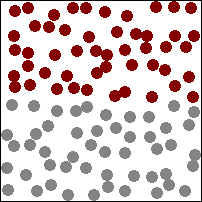The higher the temperature the more the particles vibrate and faster they move in the material storing energy.

The Thermal Energy Store is the Energy Store associated with the temperature of an object.

### About The Thermal Energy Store

The hotter an object the more energy in the thermal energy store.
The more mass an object has the more energy in its thermal energy store.
Different materials can store different amounts of energy at the same temperature.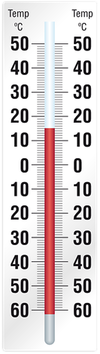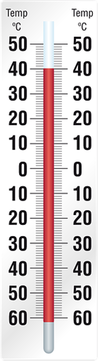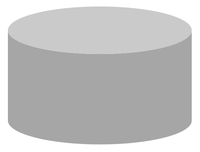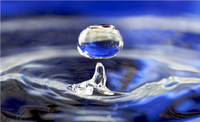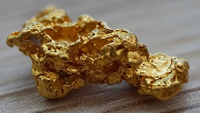### Examples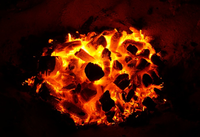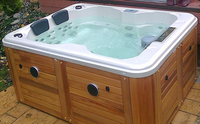The sparkler is at the highest temperature but it has the least thermal energy because it has so little mass. These embers are much colder than a sparkler but have much more mass so they have more thermal energy. The water in this hot tub is colder than both the sparkler and embers, but it has the most thermal energy because it has the most mass.

## Key Stage 4

### Meaning

The Thermal Energy Store is the energy store associated with an objects temperature which is itself due to the random motion of particles within an object.

### About the Thermal Energy Store

Thermal Energy is linked to the kinetic energy of the randomly moving particles inside an object.
The thermal energy store of an object is related to three properties of the object:

### Equation

NB: You will be given this equation in the formula sheet.

Thermal Energy = (Mass) x (Specific Heat Capacity) x (Change in Temperature)

$$E_t = m c \Delta \theta$$

Where:

Et = Thermal Energy transferred to the object.

m = Mass of the object

c = Specific Heat Capacity of the material which makes up the object

Δθ = Change in temperature of the object

### Calculating Thermal Energy

 A 2 kg object made from a material with specific heat capacity 5 J/kg/°C is heated by 10°C. Calculate the Thermal Energy transferred to the object. A sealed metal can containing 5.5 kg of water is heated over a fire from 27°C to 100°C to sterilise the water. Calculate the increase in thermal energy of the water. The Specific Heat Capacity of water is 4200 J/kg/°C. (Give your answer correct to 2 significant figures.) A 0.5kg Iron baking tray is heated from 20°C to 80°C in an oven. Calculate the work done by the oven in heating the tray. The Specific Heat Capacity of Iron is 450 J/kg/°C. (Give your answer correct to 2 significant figures.) 1. State the known quantities m = 2 kg c = 5 J/kg/°C Δθ = 10°C 1. State the known quantities m = 5.5 kg c = 4200 J/kg/°C θ1 = 27°C θ2 = 100°C Find the temperature change. Δθ = θ2 - θ1 = 100 - 27 = 73°C 1. State the known quantities m = 0.5 kg c = 450 J/kg/°C θ1 = 20°C θ2 = 80°C Find the temperature change. Δθ = θ2 - θ1 = 80 - 20 = 60°C 2. Substitute the numbers into the equation and solve. $$E_t = m c \Delta \theta$$ $$E_t = 2 \times 5 \times 10$$ $$E_t = 100J$$ 2. Substitute the numbers into the equation and solve. $$E_t = m c \Delta \theta$$ $$E_t = 5.5 \times 4200 \times 73$$ $$E_t = 1686300J$$ $$E_t \approx 1700000J$$ 2. Substitute the numbers into the equation and solve. $$E_t = m c \Delta \theta$$ $$E_t = 0.5 \times 450 \times 60$$ $$E_t = 13500J$$ $$E_t \approx 14000J$$